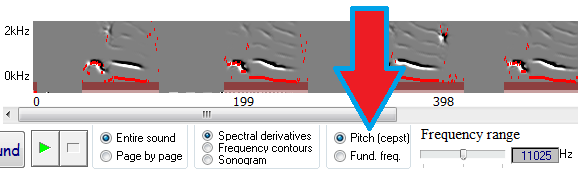# Fundamental frequency

 < PREVIOUS: Peak frequency > NEXT: Goodness of pitch Fundamental frequency is an estimate of the periodicity of the sound. In the power spectrum it is the lowest common denominator of the harmonic peaks. SAP2011 offers two methods for computing it, to select in Explore & Score, use this radio box:- Pitch: a measure used in previous versions of Sound Analysis, is a combo-measure of harmonic pitch estimate (cepstrum) and mean frequency. The cepstrum peak is usually calculated upon the spectrum of log spectrum, but instead of log spectrum we use the derivative spectrum. At any given time window, pitch might be either harmonic, sinusoidal (whistle) or not well-defined. In the two later cases, mean frequency provides an appropriate pitch estimate. Hence, at time window t, we calculate pitch according to three threshold parameters T1, T2, T3. - ff t =period where FFT(spectral_derivatives t) is the highest. (this is our cepstrum pitch estimate), and then: if { ff t > T1 || (Wiener entropy < T2 & Goodness of pitch